2022 Facts and Factors

Today’s Puzzle:

Solve these two multiplication problems and see how mirror-like they are!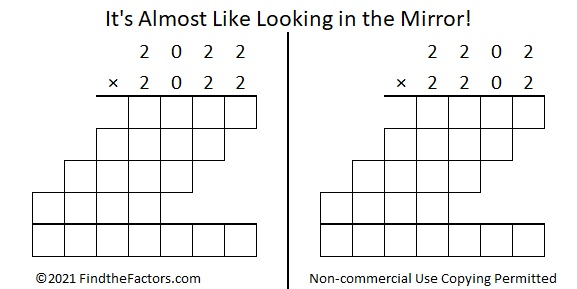Only 50 numbers less than 10000 can make a similar claim to fame:Countdown to 2022:

Early in 2021, I found a countdown equation for 2022 that also involves its factors. A couple of weeks ago, I found another one. I can’t decide which one I like the best. Even though you can only focus on one equation at a time, the countdown will show both of them concurrently: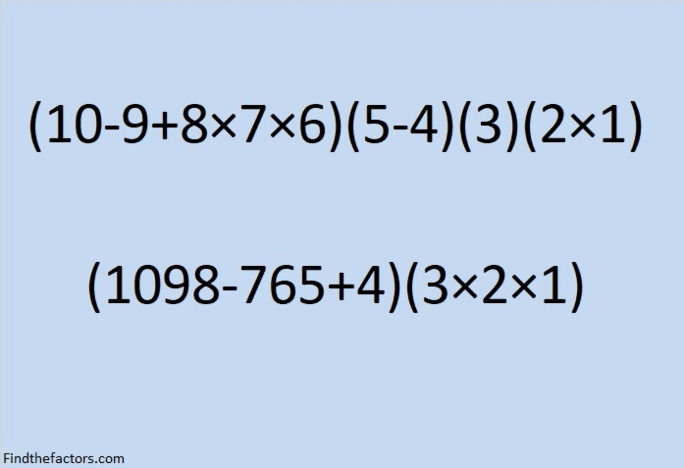make science GIFs like this at MakeaGif
Here is a still of the last frame:

Factors of 2022:

You might be asked to find the factors of 2022 several times in the coming year.
It’s an even number, so it’s divisible by 2.
It’s also easy to remember that it is divisible by 3 because 2 + 2 + 2 = 6, a multiple of 3.
This graphic may help you remember that it is divisible by 337:
• 2022 is a composite number.
• Prime factorization: 2022 = 2 × 3 × 337.
• 2022 has no exponents greater than 1 in its prime factorization, so √2022 cannot be simplified.
• The exponents in the prime factorization are 1, 1, and 1. Adding one to each exponent and multiplying we get (1 + 1)(1 + 1)(1 + 1) = 2 × 2 × 2 = 8. Therefore 2022 has exactly 8 factors.
• The factors of 2022 are outlined with their factor pair partners in the graphic below.

2022 is in the hypotenuse of a Pythagorean triple:
1050-1728-2022, which is 6 times (175-288-337).

It is also the short leg in three other Pythagorean triples:
2022-2696-3370, which is (3-4-5) times 674,
2022-340704-340710, which is 6 times (337-56784-56785), and
2022-1022120-1022122, which is 2 times (1011-511060-511061)

2022 uses only 2’s and 0’s in base 10 and in base 3:
2022₁₀ = 2202220₃.

2022 is the sum of positive consecutive numbers in three different ways: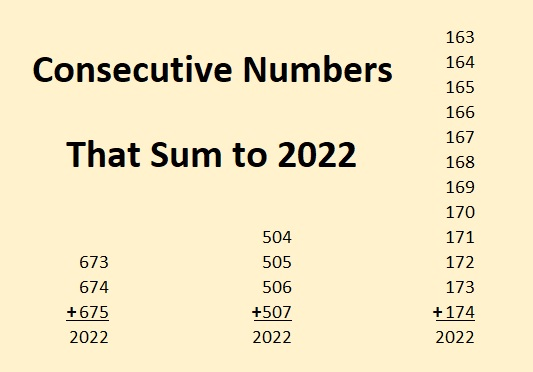2022 Magic Squares: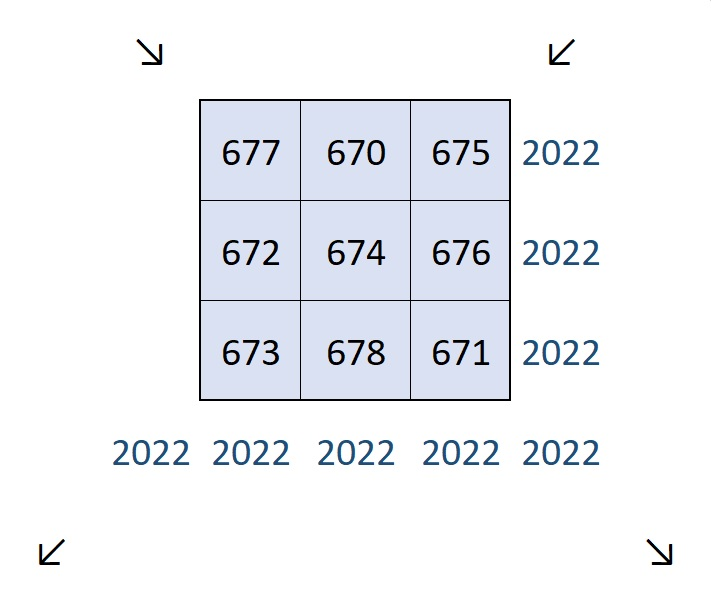All of the above are the facts that I came up with. Lots of people on Twitter have found other facts about 2022. Be sure to check them out!

Tweets Celebrating 2022’s Mathematical Properties:

Here are some tweets about 2022 that I’ve seen on Twitter. I’ll add more as I see them. They are not listed in order of difficulty, but more or less, in the order that I saw them.

I’ll end with this thread that you’ll just have to click on to appreciate. It’s packed with tons of mathematical reasons 2022 will a fabulous year!

Factors and Facts for the Year 2021

Countdown to 2021:

Here is a countdown you can use on New Year’s Eve to bring in 2021:make science GIFs like this at MakeaGif

It’s Almost Like 2021 Is Looking in a Mirror!

Squaring means multiplying a number by itself. Something unusual happens when you square 2021 and you also square its reverse, 1202. Complete the two multiplication problems below to see for yourself!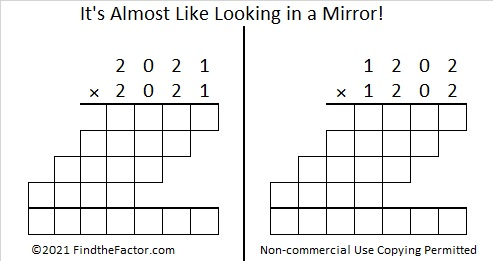There are only 50 numbers less than 10000 with this same property. They are all listed in the table below:For each pair of numbers in the table, the smaller number was listed first, but if you look carefully you will notice 2001, 2011, 2012, 2021, and 2022 in this century and 2101, 2102, 2111, and 2121 in the next.

Math Facts about the Number 2021:make science GIFs like this at MakeaGif

2021 is the difference of two squares in two different ways:
45² – 2² = 2021 and
1011² – 1010² = 2021.

2021 is not the hypotenuse of any Pythagorean triples, but it is a leg in four:
180-2021-2029, calculated from 2(45)(2), 45² – 2², 45² + 2²,
2021-43428-43475, which is (43-924-925) times 47,
2021-47472-47515, which is 43 times (47-1104-1105)
2021-2042220-2042221, from 1011² – 1010², 2(1011)(1010), 1011² + 1010².Factors of 2021:

The previous year had many negative factors, although it had just as many positive ones. I can predict that 2021 will have only four negative factors to go along with its four positive ones. I think we will see a lot of the negative factors at the beginning of the year, but hopefully, the positive ones will be more evident as the year goes on.

• 2021 is a composite number.
• Prime factorization: 2021 = 43 × 47.
• 2021 has no exponents greater than 1 in its prime factorization, so √2021 cannot be simplified.
• The exponents in the prime factorization are 1 and 1. Adding one to each exponent and multiplying we get (1 + 1)(1 + 1) = 2 × 2 = 4. Therefore 2021 has exactly 4 factors.
• The factors of 2021 are outlined with their factor pair partners in the graphic below.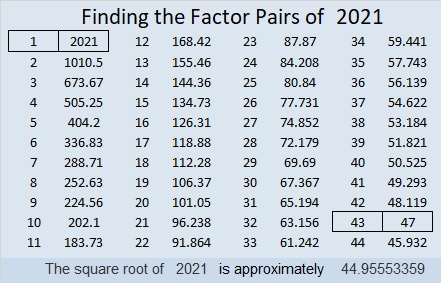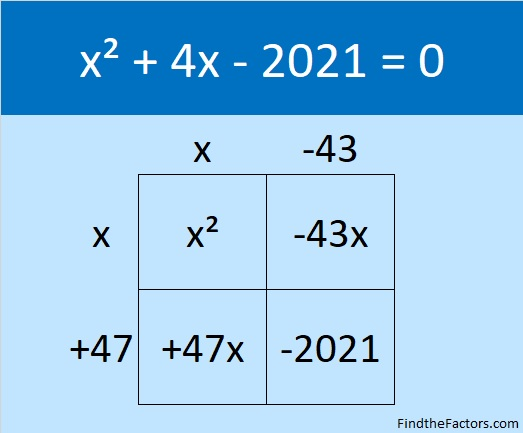Here are tweets about the number 2021 in the order that I’ve seen them. If I see more, I’ll add more.

Facts about and Factors of 2019

Here’s a countdown you can use to ring in the New Year: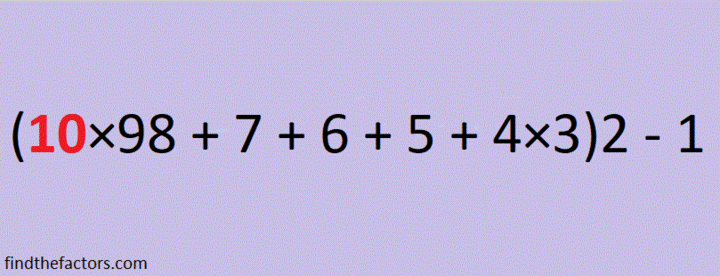make science GIFs like this at MakeaGif

2019 is the sum of consecutive numbers three different ways:
1009 + 1010 = 2019
672 + 673 + 674 = 2019
334 + 335 + 336 + 337 + 338 + 339 = 2019

There is one way that 2019 is the sum of consecutive odd numbers:
671 + 673 + 675 = 2019

2019 is the difference of two squares two different ways:
338² – 335² = 2019
1010² – 1009² = 2019

2019 is the sum of three squares nine different ways:
43² + 13² + 1² = 2019
43² + 11² + 7² = 2019
41² + 17² + 7² = 2019
41² + 13² + 13² = 2019
37² + 25² + 5² = 2019
37² + 23² + 11² = 2019
37² + 19² + 17² = 2019
35² + 25² + 13² = 2019
31² + 23² + 23² = 2019

2019 is the hypotenuse of a Pythagorean triple:
1155-1656-2019 so 1155² + 1656² = 2019²

2¹⁰ + 2⁹ + 2⁸ + 2⁷ + 2⁶ + 2⁵ + 2¹ + 2⁰ = 2019

2019 is a palindrome in a couple of bases:
It’s 5B5 in BASE 19 (B is 11 base 10) because 5(19²) + 11(19) + 5(1) = 2019,
and 3C3 in BASE 24 (C is 12 base 10) because 3(24²) + 12(24) + 3(1) = 2019

Every year has factors that often catch people by surprise. Today I would like to give you my predictions for the factors of 2019:
2019 will have four positive factors: 1, 3, 673, and 2019
However, 2019 will also have four negative factors: -1, -3, -673, and -2019

Which factors, positive or negative, will be your focus in the coming year?Finally, I’ll share some mathematics-related 2019 and New Year tweets that I’ve seen on twitter. Some of these tweets have links that contain even more facts about the number 2019.

That tweet inspired me to make my own 3 × 3 Magic Square where every number is different but every row, column and diagonal totals the same number:
But because 2019 is divisible by 3, it can also be a magic sum of 3 × 3 magic square:5

5

And finally, here is my contribution to 2019 twitter:

1009, a Prime Factor of the Year 2018

Let’s begin with a mathematical equation you can use to countdown the final seconds of 2017 to welcome in the New Year, 2018.make misc GIFs like this at MakeaGif

*********

Okay, that equation had a lot of parentheses which made us multiply by 1 two different times. (Boring.) I designed it the way it is because I wanted to take advantage of the fact that 1009 × 2 = 2018.

This second equation created by Edmark M. Law needs no parentheses and is much more beautiful: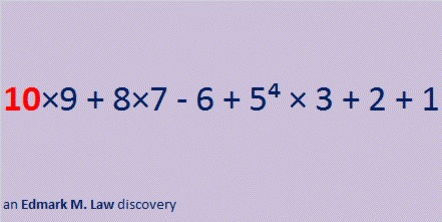make misc GIFs like this at MakeaGif

**********

Sometimes you might need a still picture instead of a gif.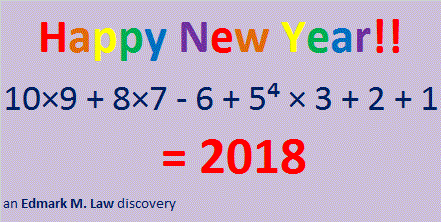This post will include lots of facts about 2018.

2018¹⁰⁻⁹⁻⁸⁺⁷⁺⁶⁻⁵⁻⁴⁺³⁺²⁻¹ = 2018

2018 can be written as the sum of four consecutive numbers:
503 + 504 + 505 + 506 = 2018

It can also be written as the sum of two consecutive even numbers:
1008 + 1010 = 2018

2018 will be an amazing year in many different areas:That graphic is based on 43² +  13² = 2018.

This next one is based on the fact that 2(1009) = 2(28² +  15²) = 2018.2018 is also the sum of three squares four different ways:

36² +  19² +  19² = 2018
35² +  28² +  3² = 2018
35² +  27² +  8² = 2018
33² +  23² +  20² = 2018

This next area problem is based on one of the sums of three squares listed above. Can you tell which one?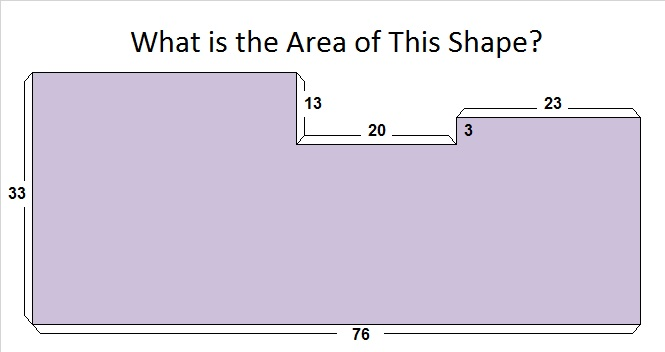2018 is in exactly two Pythagorean triple triangles:
1118-1680-2018 and 2018-1018080-1018082.In the triangle illustrated above, 2018 is about 500 times smaller than either of the other two sides. Yep, that graphic was definitely not drawn to scale.

By contrast, in this next triangle, hypotenuse 2018 is not even twice as big as either of the legs.How did I find that triangle?
1118-1680-2018 can be calculated from 2(43)(13), 43² – 13², 43² + 13²
It is also 2 times (559-840-1009). That primitive triple can be calculated from
28² – 15², 2(28)(15), 28² + 15²

Is there any other relationship between 43² + 13² and 2(28² + 15²)? Yes.
28 + 15 = 43 and 28 – 15 = 13.

How did I find the triple with two sides in the millions (2018-1018080-1018082)?
2018 ÷ 2 = 1009 and 1009² ± 1 are the values of the other leg and the hypotenuse.

I like the way 2018 looks in these other bases:
It’s 8E8 in BASE 15 (E is 14 base 10) because 8(15²) + 14(15) + 8(1) = 2018,
2G2 in BASE 28 (16 is G base 10) because 2(28²) + 16(28) + 2(1) = 2018, and
2202202 in BASE 3 because 2(3⁶ + 3⁵ + 3³ + 3² +3⁰) = 2(1009) = 2018

This is how we can write 2018 as the sum of powers of 2:
2¹⁰ + 2⁹ + 2⁸ + 2⁷ + 2⁶ + 2⁵ + 2¹ = 2018

Finally, I give you my predictions of the factors we will see in 2018. You can be confident that these predictions will be 100% correct.
The positive factors for the year 2018 will be (drum roll) 1, 2, 1009, and 2018.
Sorry to say, but there will also be four negative factors of 2018: -1, -2, -1009, and -2018.

I also know that 2018 will have some complex factors because 43² +  13² = 2018.

Here is a graphic showing 2018’s factor pairs:Related Articles:

1. Edmark M. Law’s post titled Happy New Year 2018! (And Mathematical Facts about 2018) has many more mathematical curiosities about 2018.
2. Mathwithbaddrawings.com humorously shares some upcoming mathematical dates and other facts about 2018 in Things to Know About the Year 2018.  At least one of those facts makes the number 2018 quite unique.
3. 2018: Top Ten Facts about the New Year has a little bit of mathematics in it.

Since this is my 1009 post, I’ll tell you a few things about that number:

1009 is half of 2018.

1009 is the smallest four-digit prime number.

28² + 15² = 1009 so we get this Pythagorean triple:
559-840-1009

1009 is a palindrome or otherwise looks interesting in some other bases:
It’s 838 in BASE 11 because 8(121) + 3(11) + 8(1) = 1009,
474 in BASE 15 because 4(15²) + 7(15) + 4(1) = 1009,
321 in BASE 18 because 3(18²) + 2(18) + 1(1) = 1009,
2F2 in BASE 19 (F is 15 base 10) because 2(19²) + 15(19) + 2(1) = 1009,
1I1 in BASE 24 (I is 18 base 10) because 1(24²) + 18(24) + 1(1) = 1009, and
181 in BASE 28 because 1(28²) + 8(28) + 1(1) = 1009

• 1009 is a prime number.
• Prime factorization: 1009 is prime.
• The exponent of prime number 1009 is 1. Adding 1 to that exponent we get (1 + 1) = 2. Therefore 1009 has exactly 2 factors.
• Factors of 1009: 1, 1009
• Factor pairs: 1009 = 1 × 1009
• 1009 has no square factors that allow its square root to be simplified. √1009 ≈ 31.76476

How do we know that 1009 is a prime number? If 1009 were not a prime number, then it would be divisible by at least one prime number less than or equal to √1009 ≈ 31.8. Since 1009 cannot be divided evenly by 2, 3, 5, 7, 11, 13, 17, 19, 23, 29 or 31, we know that 1009 is a prime number.Here’s another way we know that 1009 is a prime number: Since its last two digits divided by 4 leave a remainder of 1, and 28² + 15² = 1009 with 28 and 15 having no common prime factors, 1009 will be prime unless it is divisible by a prime number Pythagorean triple hypotenuse less than or equal to √1009 ≈ 31.8. Since 1009 is not divisible by 5, 13, 17, or 29, we know that 1009 is a prime number.

Now I’ll include posts from twitter that celebrate 2018 mathematically. Some are easier to understand than others:

Be sure to click on this next one. There are MANY 2018 equations in the comments:

792 Number Facts and Factors of the Year 2017

Happy New Year, everybody!

So much of what I’ve included in this post is a bit difficult for elementary school students, but here is an area problem that they should be able to do: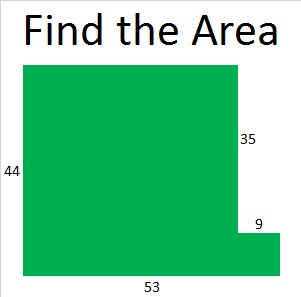Perhaps you’ll recognize that as just another way to illustrate that 44² + 9² = 2017.

This area problem based on 33² + 28² + 12² = 2017 will be a little bit more challenging for students: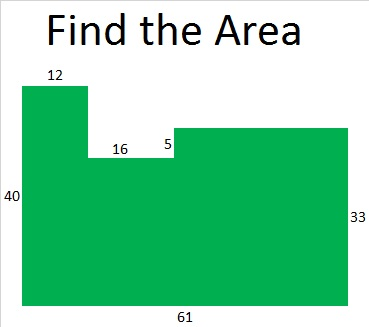2016 had more factors, positive and negative, than anybody could have imagined, but 2017 is a prime number year, so hopefully it will be filled with less drama.

I have a lot to say about the number 2017 with a little help from twitter.

2017 has only TWO positive real factors, 1 and 2017, and only TWO negative real factors, -1 and -2017. Positive or negative, ALL the real possible factor pairs for 2017 are

• 1 x 2017 and (-1) x (-2017).

2017 = 4(504) + 1. So 2017 is the sum of two squares. Which ones?

44² + 9² = 2017. That sum-of-squares number fact means that 2017 is the hypotenuse of a Pythagorean triple, specifically, 792-1855-2017. Since 2017 is a prime number, this triple is also a primitive.Here’s how those numbers were calculated from the fact that  44² + 9² = 2017:

• 2(44)(9) = 792
• 44² – 9² = 1855
• 44² + 9² = 2017

2017 is also the short leg in a rather monstrous primitive Pythagorean triple:

• 2017² + 2,034,144² = 2,034,145²

Hmm. 44² + 9² = 2017 means we can find some COMPLEX factor pairs for 2017:

• (44 + 9i)(44 – 9i) = 2017
• (-44 + 9i)(-44 – 9i) = 2017
• (9 + 44i)(9 – 44i) = 2017
• (-9 + 44i)(-9 – 44i) = 2017

Who knows what all those COMPLEX factors will bring to the coming year? Each of them was derived from the fact that 44² + 9² = 2017.

2017 is the sum of THREE squares three different ways:

• 37² + 18² + 18²  = 2017
• 33² + 28² + 12² = 2017
• 30² + 26² + 21² = 2017

Since 37² = 35² + 12², 30² = 24² + 18², and 26² = 24² + 10², we can write 2017 as the sum of these squares, too:

• 35² + 18² + 18² + 12² = 2017
• 30² + 24² + 21² + 10² = 2017
• 26² + 24² + 21² + 18² = 2017
• 24² + 24² + 21² + 18² + 10² = 2017

Here’s more sums and/or differences of squares from twitter:

This representation of 2017 is “two” powerful:

2017 is a PALINDROME in bases 31, 32, and 36:

• 232 BASE 31; note that 2(31²) + 3(31) + 2(1) = 2017
• 1V1 BASE 32 (V is 31 base 10); note that 1(32²) + 31(32) + 1(1) = 2017
• 1K1 BASE 36 (K is 20 base 10); note that 1(36²) + 20(36) + 1(1) = 2017

Also, OEIS.org tells us this 2017 fact about the totient function: “2017 is a value of n for which φ(n) = φ(n-1) + φ(n-2).”

PROOF that 2017 is a prime number:

We can use these three facts to help us verify that 2017 is a prime number:

1. 2017 = 4(504) + 1
2. 44² + 9² = 2017
3. √2017 ≈ 44.8998886

Since 2017 can be written as one more than a multiple of four, and 44 and 9 have no common prime factors, 2017 will be a prime number unless it is divisible by 5, 13, 17, 29, 37, or 41 (all the prime numbers less than √2017 ≈ 44.9 that have a remainder of one when divided by 4). That’s right, we ONLY have to divide 2017 by those SIX numbers to verify that it is prime: (Read here for why this is true.)

• 2017 ÷ 5 = 403.4
• 2017 ÷ 13 ≈ 155.15
• 2017 ÷ 17 ≈ 118.65
• 2017 ÷ 29 ≈ 69.55
• 2017 ÷ 37 ≈ 54.51
• 2017 ÷ 41 ≈ 49.20

We don’t get a whole number answer for any of those divisions, so 2017 is prime!

If you’re looking for more reasons to be interested in the number 2017, read David Radcliffe’s article:

Here are some other lovely mathematical thoughts about 2017 that I found on twitter:

In case you don’t know (and apparently a lot of people don’t know), 0! = 1, so this expression really does equal 2017.

And finally, if you click on this next link, David Mitchell will explain the tessellation of the number 2017.

——————————–

Since this is my 792nd post, I’ll write a little bit about the number 792:

792 has 24 factors so I’ll include a few of its many possible factor trees: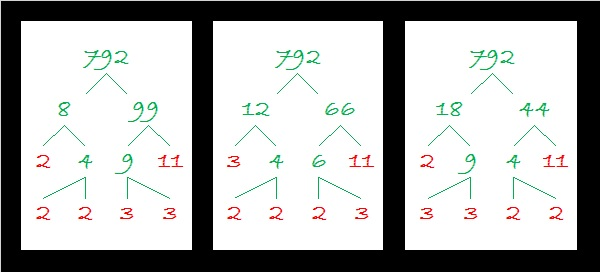Since 792 has so many factors, it is a leg in MANY Pythagorean triples. Here I list some factors that generate PRIMITIVE triples:

• 2(396)(1): 792-156815-156817
• 2(99)(4): 792-9785-9817
• 2(44)(9): 792-1855-2017 (illustrated near the top of this post)
• 2(36)(11): 792-1175-1417

792 is the sum of three squares four different ways including three ways that repeat squares:

• 28² + 2² + 2² = 792
• 26² + 10² + 4² = 792
• 20² + 14² + 14² = 792
• 18² + 18² + 12² = 792

792 is a palindrome in bases 32 and 35:

• OO BASE 32 (O is 24 base 10); note that 24(32) + 24(1) = 792
• MM BASE 35 (M is 22 base 10); note that 22(35) + 22(1) = 792

Finally here is the factoring information for the number 792:

• 792 is a composite number.
• Prime factorization: 792 = 2 x 2 x 2 x 3 x 3 x 11, which can be written 792 = (2^3) x (3^2) x 11
• The exponents in the prime factorization are 3, 2 and 1. Adding one to each and multiplying we get (3 + 1)(2 + 1)(1 + 1) = 4 x 3 x 2 = 24. Therefore 792 has exactly 24 factors.
• Factors of 792: 1, 2, 3, 4, 6, 8, 9, 11, 12, 18, 22, 24, 33, 36, 44, 66, 72, 88, 99, 132, 198, 264, 396, 792
• Factor pairs: 792 = 1 x 792, 2 x 396, 3 x 264, 4 x 198, 6 x 132, 8 x 99, 9 x 88, 11 x 72, 12 x 66, 18 x 44, 22 x 36 or 24 x 33

Taking the factor pair with the largest square number factor, we get √792 = (√36)(√22) = 6√22 ≈ 28.14249.728 Number Facts and Factors of the Year 2016

The year 2016 will have some fun mathematical properties:

2016 is the sum of all the counting numbers from 1 to 63. That makes 2016 the 63rd triangular number which can be calculated rather quickly using 63 x 64/2 = 2016. That is definitely an example of multiplication being a shortcut for addition!

When was the last time a year was a triangular number? 1953. That’s the sum of the first 62 numbers, and it is also the year that I was born.

Besides being the sum of the counting numbers from 1 to 63, 2016 is the sum of consecutive numbers a few other ways as well:

• 671 + 672 + 673 = 2016; that’s 3 consecutive numbers.
• 285 + 286 + 287 + 288 + 289 + 290 + 291 = 2016; that’s 7 consecutive numbers.
• 220 + 221 + 222 + 223 + 224 + 225 + 226 + 227 + 228 = 2016; that’s 9 consecutive numbers.
• 86 + 87 + 88 + 89 + 90 + 91 + 92 + 93 + 94 + 95 + 96 + 97 + 98 + 99 + 100 + 101 + 102 + 103 + 104 + 105 + 106 = 2016; that’s 21 consecutive numbers.

2016 is the sum of the eighteen prime numbers from 71 to 157.2016 is also the 32nd hexagonal number because 2 x 32² – 32 = 2016. (All hexagonal numbers are also triangular numbers, and half of all triangular numbers are hexagonal numbers.)

—————–

As you read this post, you may get the impression that 2016 is a perfect number. All perfect numbers are triangular numbers AND hexagonal numbers, but 2016 is still NOT a perfect number. Here’s why:

The sum of the first 31 factors of 2016 is

1 + 2 + 3 + 4 + 6 + 7 + 8 + 9 + 12 + 14 + 16 + 18 + 21 + 24 + 28 + 32 + 36 + 56 + 63 + 72 + 84 + 96 + 112 + 126 + 144 + 168 + 224 + 252 + 288 = 2016

but if you add in its other four factors 336 + 504 + 672 + 1008, you get 4536 which is way too much to make 2016 a perfect number.

True, 2016 is (2^5)(2^6 – 1) = 32 x 63, and all perfect numbers can be written similarly with consecutive powers of 2, BUT the odd factor, 63, is not a PRIME number so 2016 is not a perfect number.

IF 63 WERE a prime number, then the sum of the factors of 2016 would be

1 + 2 + 4 + 8 + 16 + 32 + 63 + 126 + 252 + 504 + 1008 = 2016

But again, 63 is NOT a prime number so 3, 6, 7, 9, 12, 14, 18, 21, 24, 28, 36, 42, 48, 56, 72, 84, 96, 112, 144, 168, 224, 288, 336, and 672 are also factors of 2016, and 2016 is NOT a perfect number.

—————–

Even though 2016 is NOT a perfect number, it is still an exciting number in many more ways:

2016 = (10 × 9 × 8 × 7 × 6)/(5 + 4 + 3 + 2 + 1)

From OEIS.org we learn that the sum of the square and cube of 2016 is a number containing all the digits 0 – 9 exactly once:2016 is the short leg in these four primitive Pythagorean triples:

• 2016-3713-4225
• 2016-12,463-12,625
• 2016-20,687-20,785
• 2016-1,016,063-1,016,065

2016 is not a palindrome in any base until base 47. We just need a symbol to represent 42 base 10 in base 47 because 42(47) + 42(1) = 2016.

Thank you Slate magazine  for including my post in an article about properties of 2016. Also thank you for referring me to eljjdx.canalblog.com which has some very interesting information about the number 2016 that I didn’t include in this post. Something on my computer did a great job translating both of those articles from French into English.

Reflexivemaths has also written many thoughtful starter questions that explore the number 2016.

Before I give all the factors we can expect to see in the year 2016, let me share a few fun number facts that I saw on twitter:

That is the most complicated use of combinations I have ever seen. The innermost parenthesis means 4!/(2!∙2!) which equals 6. Since 2 was raised to that power, we get 2^6 = 64. Then 64!/(62!∙2!) = 2016.

————————————————

The last two years on New Year’s Eve I have predicted the factors of the coming year. Each time my predictions were 100% accurate. I am now ready to make my predictions for the factors of 2016:

(Drum roll) There will be 36 positive factors for 2016:

1, 2, 3, 4, 6, 7, 8, 9, 12, 14, 16, 18, 21, 24, 28, 32, 36, 56, 63, 72, 84, 96, 112, 126, 144, 168, 224, 252, 288, 336, 504, 672, 1008, 2016. Sadly, if you multiply any of them by -1, you’ll know a negative factor for 2016, too.

The factors of 2016 will also come in pairs: 1 x 2016, 2 x 1008, 3 x 672, 4 x 504, 6 x 336, 7 x 288, 8 x 252, 9 x 224, 12 x 168, 14 x 144, 16 x 126, 18 x 112, 21 x 96, 24 x 84, 28 x 72, 32 x 63, 36 x 56and 42 x 48.Because the number of 2016’s prime factors is a power of two, 2016 can make a nicely proportioned factor tree especially if we use any of the factor pairs that are in red. (14 x 114 is in bold only because I like the way it looks.) Because 2016 has 8 prime factors, we can get a very full and impressive tree. For example:Since this is my 728th post, I’ll write a little about the number 728, too. I’ll start with this factor tree for 728: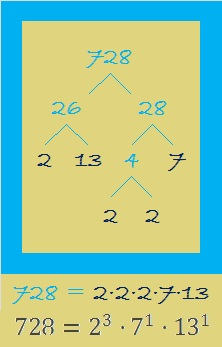It’s not as impressive as the factor tree for 2016, but did you notice that 2016 and 728 share several of the same prime factors?

When two numbers share some of the same factors, we may wonder what is the greatest common factor and what is the least common multiple?

One way to find either value is to use the prime factorization of both numbers. The prime factorization of 2016 uses three bases: 2, 3, and 7, while the prime factorization for 728 uses these bases: 2, 7, and 13. Write down all those bases without duplication, and you get 2, 3, 7, and 13.

For the GREATEST COMMON FACTOR (GCF), look at the prime factorizations and choose the SMALLEST exponent that appears in each. The greatest common factor cannot be bigger than the smallest number, 728. In this case it is 56 which will divide evenly into both 728 and 2016.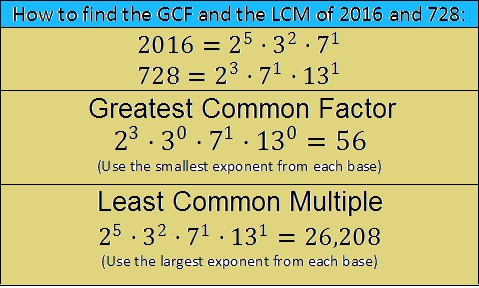For the LEAST COMMON MULTIPLE (LCM), look at the prime factorizations and choose the LARGEST exponent that appears in each. The least common multiple cannot be smaller than the largest number, 2016. In this case it is 26,208 which both 728 and 2016 can divided into evenly.

This method for finding the GCF and LCM will also work for three, four, or more numbers and even variable bases like x, y, or z. I encourage you to give it a try!

Here are some more number facts about the number 728:

26 x 28 = 728, and both 26 and 28 are one number away from 27, their average, so 728 is one number away from 27².

Here’s proof: 26 x 28 = (27 – 1)(27 + 1) = (27² – 1²) = 729 – 1 = 728.

Because 13 is one of its prime factors, 728 is the hypotenuse of Pythagorean triple 280-672-728. The greatest common factor of those three numbers is the same as the greatest common factor of 728 and 2016, but what is their least common multiple? It turns out to be 5 x 12 x 13 x 56, the product of the numbers in the primitive Pythagorean triple, 5-12-13, and 56. Using prime factorizations we get (2^5)(3^1)(5^1)(7^1)(13^1). We get the same answer using either method.

728 is a palindrome (repdigit) in three other number bases:

• 728 is 222222 BASE 3; note that 2(3^5) + 2(3^4) + 2(3^3) + 2(3^2) + 2(3^1) + 2(3^0) = 728.
• 888 BASE 9; note that 8(81) + 8(9) + 8(1) = 728.
• QQ BASE 27 (Q = 26 base 10); note that 26(27) + 26(1) = 728.

Here is more factoring information for 728:

————————————————

• 728 is a composite number.
• Prime factorization: 728 = 2 x 2 x 2 x 7 x 13, which can be written 728 = (2^3) x 7 x 13
• The exponents in the prime factorization are 1, 3, and 1. Adding one to each and multiplying we get (1 + 1)(3 + 1)(1 + 1) = 2 x 4 x 2 = 16. Therefore 728 has exactly 16 factors.
• Factors of 728: 1, 2, 4, 7, 8, 13, 14, 26, 28, 52, 56, 91, 104, 182, 364, 728
• Factor pairs: 728 = 1 x 728, 2 x 364, 4 x 182, 7 x 104, 8 x 91, 13 x 56, 14 x 52, or 26 x 28
• Taking the factor pair with the largest square number factor, we get √728 = (√4)(√182) = 2√182 ≈ 26.981475.————————————————

337 What Will Be the Factors of 2015?

Since this is my 337th post, I’ll first give some information about the number 337, then I will predict the factors for 2015.

• 337 is a prime number.
• Prime factorization: 337 is prime.
• The exponent of prime number 337 is 1. Adding 1 to that exponent we get (1 + 1) = 2. Therefore 337 has exactly 2 factors.
• Factors of 337: 1, 337
• Factor pairs: 337 = 1 x 337
• 337 has no square factors that allow its square root to be simplified. √337 ≈ 18.358How do we know that 337 is a prime number? If 337 were not a prime number, then it would be divisible by at least one prime number less than or equal to √337 ≈ 18.358. Since 337 cannot be divided evenly by 2, 3, 5, 7, 11, 13, or 17, we know that 337 is a prime number.

Celebrate the New Year by giving this puzzle a try!

Print the puzzles or type the factors on this excel file: 12 Factors 2014-12-29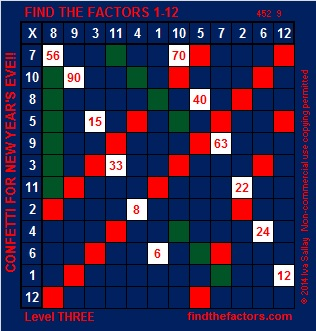On New Year’s Eve 2013 I predicted that the positive factors for 2014 would be 1, 2, 19, 38, 53, 106,1007, and 2014, and my predictions were 100% accurate!

On this last day of 2014, I boldly announce my predictions for the factors of the year 2015:

• The positive factors for 2015 will be 1, 5, 13, 31, 65, 155, 403, and 2015
• Some of these factors will occur in pairs: 1 and 2015, 5 and 403, 13 and 155, as well as 31 and 65.
• Unfortunately there will be some negative factors in 2015 as well. They will be -1, -5, -13, -31, -65, -155, -403, and -2015.

Whatever life throws your way, I wish you a happy, healthy, and prosperous 2015.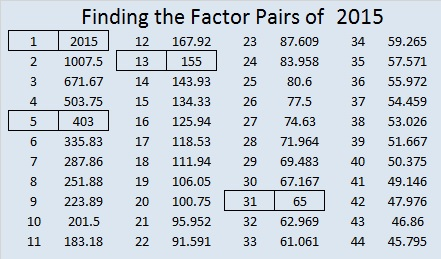21 Factors of the Year 2013 and 2014

21 is a composite number. 21 = 1 x 21 or 3 x 7. Factors of 21: 1, 3, 7, 21. Prime factorization: 21 = 3 x 7.When 21 is a clue in the FIND THE FACTORS puzzles, use 3 x 7.

Scroll down the page to find factoring information about 2013 and 2014.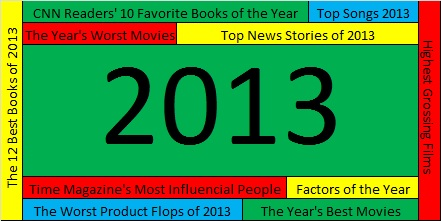Near the end of each year movie critics make lists of the ten best movies and the ten worse movies of the year. News agencies list the ten most significant news stories. Time magazine lists the ten most influential people of the year. The music industry lists the top ten songs of the year. As 2013 draws to a close, it is most appropriate for me to review the factors of the year.

2013 had exactly 8 positive factors. These factors were 1, 3, 11, 33, 61, 183, 671, and 2013.

There is no room for argument. I am absolutely certain this list is complete. No one will make any comments disagreeing with me, calling me names, or asking how I could have left Two or Five or Seven off the list. Also no one will wonder why I would include forgettable 671 on the list. Do the Math. 671 was clearly a factor in 2013. Three of the factors of 2013 were also prime factors. They were 3, 11, and 61. This graphic clearly shows those prime factors.2013 also had 8 negative factors. The first negative factor on the list is no surprise: Minus One. Year in and year out we can count on Minus One being a negative factor. Some other factors were just as negative in 2013, namely -3, -11, -33, – 61, -183, -671, and -2013. Of course, many of those factors were so obscure that most people never gave them a second thought all year long. Again I expect no arguments or negative comments on these selections. Anyone who knows anything about factors will have to agree with this list.

Even though 2014 hasn’t even started, I am going to predict the factors of 2014, and I am absolutely positive that my predictions will be 100% correct. You will not even have to wait until the end of 2014 to verify my accuracy.

The positive factors of 2014 will be (drum roll) 1, 2, 19, 38, 53, 106,1007, and 2014.

Most people expect the number One to be a positive factor every single year, and it will not let us down in 2014. The number Two has a reputation of being a factor only about half the time. Since she was not a factor at all in 2013, I am confident that she will get her act together again in 2014 and become a factor once more. All the other factors I’ve listed have not been factors for a very long time, and each one of them is due to make a difference over and over again in 2014 until they have nothing leftover. I predict that 2014 will have three prime factors, namely 2, 19, and 53, as illustrated in the following graphic.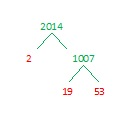How can I make such accurate assessments and spot on predictions? I will tell you: I work with factors almost every single day, and I’ve spent years observing them. Every time I have been given an assignment to become acquainted with them, I have approached that assignment with enthusiasm and determination.

Regardless of my astounding record, YOU can become just as much an expert as I am with just a little bit of knowledge and effort. You may discover, as I have, that factoring can be great fun. Here are a couple of logic puzzles that require factoring to solve:

All you have to do to solve one of the puzzles is write the numbers 1 – 12 in the top row and again in the first column so that those numbers are the factors of the given clues. Each puzzle has only one solution.

At the top of this post is a page titled How to Find the Factors, and it gives hints to solve the puzzles.   Click 12 Factors 2013-12-30 to find a printable version of these and a few other puzzles as well as the solutions for last week’s puzzles. Excel or comparable spreadsheet program is needed to open the file.

Have a great 2014 and happy factoring!

Related Articles